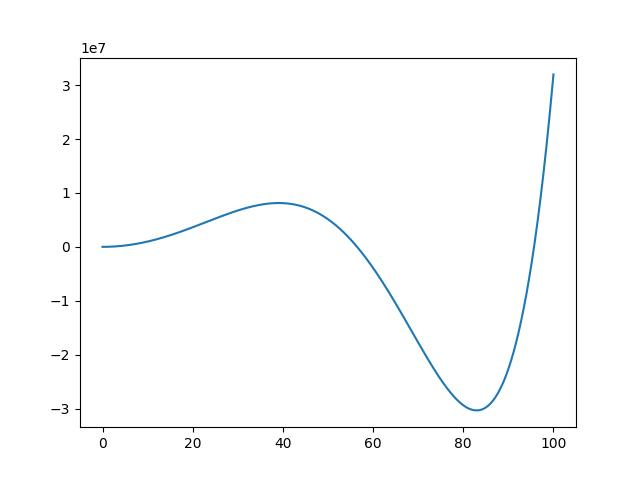# 二維

import numpy as np
import pylab as plt
x=np.linspace(0,100, 10000000)
y=0.001*(x**6)-0.1*(x**5)-0.68*(x**4)+10000*(x**2)+2
plt.plot(x,y)
plt.show()# 三維

$z=ax^4+by^4+cx^3+dy^3+ex^2+fy^2+gx+hy+i$。

ps : a, b, c, d, e, f, g, h, i  是常數

import plotly.graph_objs as go
import numpy as np
import pandas as pd
import plotly
z=np.zeros([100,100])
for x in range(0,100):
for y in range(0, 100):
z[x, y]=(0.0006*x**6-0.005*y**6+0.5*x**5-0.1*y**5+0.005*x**4+0.003*y**4)/10000000
df=pd.DataFrame(data=z)
trace=go.Surface(z=df.values) # 將dataframe所有的值以list列出
data=[trace]
layout=go.Layout(title='3D', autosize=True,
margin=dict(l=50, r=50, b=50, t=50))
fig = go.Figure(data=data, layout=layout)
fig.show()
#plotly.offline.plot(fig, filename="3d.html", auto_open=True)


# 多維

$y=ax_{1}^5+bx_{2}^5+cx_{3}^5+….$